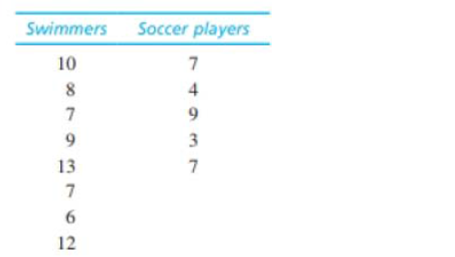Chapter 10, Problem 23PEssentials of Statistics for the B...

8th Edition
Frederick J Gravetter + 1 other
ISBN: 9781133956570

Solutions

Chapter
SectionEssentials of Statistics for the B...

8th Edition
Frederick J Gravetter + 1 other
ISBN: 9781133956570
Textbook Problem

Downs and Abwender (2002) evaluated soccer players and swimmers to determine whether the routine blows to the head experienced by soccer players produced long-term neurological deficits. In the study, neurological tests were administered to mature soccer players and swimmers and the results indicated significant differences. In a similar study, a researcher obtained the following data.a. Are the neurological test scores significantly lower for the soccer players than for the swimmers in the control group? Use a one-tailed test with α = .05.b. Compute the value of r2 (percentage of variance accounted for) for these data.

a.

To determine

To find: Whether the neurological test scores are significantly lower for the soccer player than for the swimmers using one-tailed test with α=0.05

Explanation

Given info:

Two samples each of size 8 (for swimmers) and 5 (for soccer players). One-tailed hypothesis test using α=0.05 is to be performed.

Calculations:

Step 1: Null Hypothesis is H0:μ1μ2 and Alternate Hypothesis is H1:μ1>μ2

Step 2: For the given sample, the t-statistics will have degrees of freedom equals:

df=n1+n22=8+52=11

For a one-tailed test with α=0.05 and df=11, the critical value (CV) is obtained from the t-table as t=1.796.

Step 3: t-statistics is calculated using formula:

t=(M1M2)(μ1μ2)sM1M2

The values of sample means, and variance are obtained below:

Software procedure:

Step-by-step procedure to obtain the sample mean and variance for the two groups, using the SPSS software:

• Go to Variable View> Enter the name of the variable as Swimmers and Soccer.
• Go to Data View>Enter the values of Swimmers and Soccer.
• Choose Analyze > Descriptive Statistics> choose Descriptive.
• Select Swimmers and Soccer and move it under variable(s)> Choose Options> Select Mean , Std. Deviation and Variance
• Choose Continue> choose OK.

Output using the SPSS software is given below:

From the SPSS output, the value sample means, and variance are

M1=9,M2=6,s12=6.28,s22=6 Respectively.

Pooled variance:

sp2=(df1)s12+(df2)s22df1+df2=(7)6

b.

To determine

To find: The value of r2 for the given question.

Still sussing out bartleby?

Check out a sample textbook solution.

See a sample solution

The Solution to Your Study Problems

Bartleby provides explanations to thousands of textbook problems written by our experts, many with advanced degrees!

Get Started

How much work is done when a hoist lifts a 200-kg rock to a height of 3 m?

Single Variable Calculus: Early Transcendentals, Volume I

Find g(1)+g(2)+g(3)+....+g(50), given that g(x)=124x.

Finite Mathematics for the Managerial, Life, and Social Sciences

Expand each expression in Exercises 122. x(4x+6)

Finite Mathematics and Applied Calculus (MindTap Course List)

Use series to evaluate the following limit. limx0sinxxx3

Single Variable Calculus: Early Transcendentals

The graph of x = 2 + 3t, y = 4 − t is a: circle ellipse line parabola

Study Guide for Stewart's Single Variable Calculus: Early Transcendentals, 8th